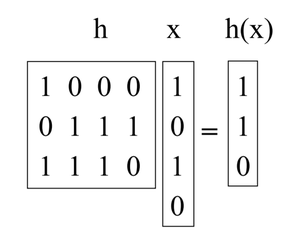Open in App
Not now

# Introduction to Universal Hashing in Data Structure

• Last Updated : 19 Oct, 2021

Hashing is a great practical tool, with an interesting and subtle theory too. In addition to its use as a dictionary data structure, hashing also comes up in many different areas, including cryptography and complexity theory

This article discusses an important notion: Universal Hashing (also known as universal hash function families).

Universal Hashing refers to selecting a hash function at random from a family of hash functions with a certain mathematical property. This ensures a minimum number of collisions.

A randomized algorithm H for constructing hash functions h : U → {1,… ,M} is universal if for all (x, y) in U such that x ≠ y, Pr h∈H [h(x) = h(y)] ≤ 1/M (i.e, The probability of x and y such that h(x) = h(y) is <= 1/M for all possible values of x and y).

A set H of hash functions is called a universal hash function family if the procedure choose h ∈ H at random is universal. (Here the key is identifying the set of functions with the uniform distribution over the set.)

Theorem: If H is a set of the universal hash function family, then for any set S ⊆ U of size N, such that x ∈ U and y ∈ S, the expected number of collisions between x and y is at most N/M.

Proof: Each y ∈ S (y ≠ x) has at most a 1/M chance of colliding with x by the definition of “universal”. So,

• Let Cxy = 1 if x and y collide and 0 otherwise.
• Let Cx denote the total number of collisions for x. So, Cx = ∑ y∈S, x≠y Cxy.
• We know E[Cxy] = Pr[ x and y collide ] ≤ 1/M.
• So, by the linearity of expectation, E[Cx] = ∑y E[Cxy] < N/M.

Corollary: If H is a set of the universal hash function family, then for any sequence of L insert, lookup, or delete operations in which there are at most M elements in the system at any time, the expected total cost of the L operations for a random h ∈ H is only O(L) (viewing the time to compute h as constant).

For any given operation in the sequence, its expected cost is constant by the above theorem. Therefore, the expected total cost of the L operations is O(L) by the linearity of expectation.

Constructing a universal hash family using the matrix method:

Let’s say keys are u-bits long and the table size M is the power of 2, so an index is b-bits long with M = 2b.

What we will do is pick h to be a random b-by-u binary matrix, and define h(x) = hx, where hx is calculated by adding some of the columns of h (doing vector addition over mod 2) where the 1 bits in x indicate which columns to add. (e.g., the 1st and 3rd columns of h are added in the below example). These matrices are short and fat. For instance:Now, take an arbitrary pair of keys (x, y) such that x ≠ y. They must differ someplace, let’s assume they differ in the ith coordinate, and for concreteness say xi = 0 and yi = 1. Imagine we first choose all of h but the ith column. Over the remaining choices of the ith column, h(x) is fixed. However, each of the 2b different settings of the ith column gives a different value of h(y) (in particular, every time we flip a bit in that column, we flip the corresponding bit in h(y)). So there is exactly a 1/2b chance that h(x) = h(y).

My Personal Notes arrow_drop_up